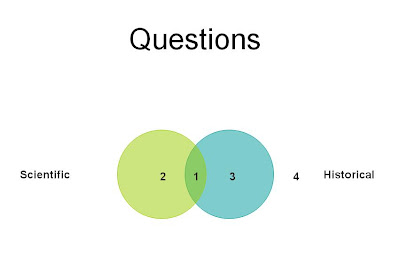# A Scientific Question? Part 8There are four different possible kinds of questions, in relation to the categories of “scientific questions” and “historical questions” (prior to doing an analysis of these concepts):
X.S…..H
1. T…..T
2. T…..F
3. F…..T
4. F…..F

(1) both scientific and historical
(2) scientific but not historical
(3) not scientific but historical
(4) not scientific and not historical

I am going to make a few plausible assumptions about what sorts of questions exist:

(a) There are some scientific questions.
(b) There are some historical questions.
(c) There are some questions that are niether scientific nor historical (such as moral questions, e.g. “Is it always wrong to to tell a lie?”).

Based on these assumptions there are five logical possibilities for the distribution of questions in relation to the four categories of questions.

Zero categories/regions have instances.
This possibility is ruled out by the assumptions above.

One category/region has instances.
This possibility is ruled out by the assumptions above.

Two categories/regions have instances.
There is only one possible distribution of questions of this sort, given the above assumptions.Three categories/regions have instances.
There are three possible distributions of questions of this sort, given the above assumptions. One of these possible distributions corresponds to a NOMA (non-overlapping magisteria) type of view about the relationship of science to history:

Four Categories/Regions have an instance.
There is just one possible distribution of questions of this sort: